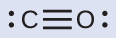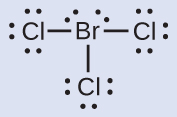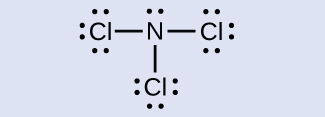# 7.4 Formal charges and resonance

 Page 1 / 9
By the end of this section, you will be able to:
• Compute formal charges for atoms in any Lewis structure
• Use formal charges to identify the most reasonable Lewis structure for a given molecule
• Explain the concept of resonance and draw Lewis structures representing resonance forms for a given molecule

In the previous section, we discussed how to write Lewis structures for molecules and polyatomic ions. As we have seen, however, in some cases, there is seemingly more than one valid structure for a molecule. We can use the concept of formal charges to help us predict the most appropriate Lewis structure when more than one is reasonable.

## Calculating formal charge

The formal charge    of an atom in a molecule is the hypothetical charge the atom would have if we could redistribute the electrons in the bonds evenly between the atoms. Another way of saying this is that formal charge results when we take the number of valence electrons of a neutral atom, subtract the nonbonding electrons, and then subtract the number of bonds connected to that atom in the Lewis structure.

Thus, we calculate formal charge as follows:

$\text{formal charge}=\text{# valence shell electrons (free atom)}-\text{# lone pair electrons}\phantom{\rule{0.2em}{0ex}}-\phantom{\rule{0.2em}{0ex}}\frac{1}{2}\phantom{\rule{0.2em}{0ex}}\text{# bonding electrons}$

We can double-check formal charge calculations by determining the sum of the formal charges for the whole structure. The sum of the formal charges of all atoms in a molecule must be zero; the sum of the formal charges in an ion should equal the charge of the ion.

We must remember that the formal charge calculated for an atom is not the actual charge of the atom in the molecule. Formal charge is only a useful bookkeeping procedure; it does not indicate the presence of actual charges.

## Calculating formal charge from lewis structures

Assign formal charges to each atom in the interhalogen ion ${\text{ICl}}_{4}{}^{\text{−}}.$

## Solution

1. We divide the bonding electron pairs equally for all I–Cl bonds:2. We assign lone pairs of electrons to their atoms . Each Cl atom now has seven electrons assigned to it, and the I atom has eight.
3. Subtract this number from the number of valence electrons for the neutral atom:
I: 7 – 8 = –1
Cl: 7 – 7 = 0
The sum of the formal charges of all the atoms equals –1, which is identical to the charge of the ion (–1).

Calculate the formal charge for each atom in the carbon monoxide molecule:C −1, O +1

## Calculating formal charge from lewis structures

Assign formal charges to each atom in the interhalogen molecule BrCl 3 .

## Solution

1. Assign one of the electrons in each Br–Cl bond to the Br atom and one to the Cl atom in that bond:2. Assign the lone pairs to their atom. Now each Cl atom has seven electrons and the Br atom has seven electrons.
3. Subtract this number from the number of valence electrons for the neutral atom. This gives the formal charge:
Br: 7 – 7 = 0
Cl: 7 – 7 = 0
All atoms in BrCl 3 have a formal charge of zero, and the sum of the formal charges totals zero, as it must in a neutral molecule.

Determine the formal charge for each atom in NCl 3 .

N: 0; all three Cl atoms: 0How does an element differ from a compound? How are they similar?
an element is an indivisible particles that can take part in a reaction and consist of smaller or tiny particles i.e proton, neutrons and electron while a compound is when two or more element chemically combine together. They are similar when they are homogeneous compound. they take the same rxn.
Yusuf
How to get the Lewis formula of SeCl+3
hi,I'm new here can I join the conversation
EZEA
what is the structural formula for starch
Starch is a mixture (of chemicals) of amylose and amylopectin. Both are macromolecules and polymers. You can search on wikipedia.
Abdelkarim
what is the roles of filter bed
Fathmat
what is the roles of Alu m
Fathmat
what is the roles of chlorine
Fathmat
Roles can be classified or correlate it to different areas: For example: Chlorine can be used in reactions (in industry) to manufacture HCl, which then can be used for other things. Or in swimming pools to kill bacteria. Or as a component in compounds with pharmaceutical roles (drugs). For Al:
Abdelkarim
Its dentisty value is suitable to be used in alloys (mixture of metals) in aircraft bodies. Also, Aluminium foils, Tin cans,.. Some of them are also in Al overhead cables in streets and long roads.
Abdelkarim
what is chemistry
Maxamed
what is the meaning of exceedingly
it is an adverb which means extremely
Rohini
what is atomic chemistry?
Lewis structure for no3
Lewis structure for no3
what is weak acid
It is an acid which partially ionises in water.
Abdelkarim
what is incandescence
Clifton
what makes it glow
Clifton
why is it red, irange and yellow in color
Clifton
hello am new here and I want to join you
Aliyu
hello
Clifton
hi
Aliyu
too
Gillian
hello i am new here please i want to join this group
Paul
Hi, I'm also new here
Salaudeen
Hi
Keeya
hello guys !!
Sourav
what is pressure?
The force applied to suction Area of the body
Ahmed
Matter composed of exceedingly small paticle called atom.
Yushao
questions related to metals
occurrence and preparation of the representatives metals
Regina
list the 20, periodic table and their symbols
hydrogen:h helium;he lithium:l beryllium:be Boron:b Carbon;C Nitrogen:n Oxygen:O FLUORINE:f Neon:n Sodium:s Magnesium:mg Aluminum:a Silicon:s Phosphorus:p Sulphur:s Chlorine:c Argon;a Potassium:p Calcium:c
Benita
Hydrogen, helium, lithium, beryllium, boron, carbon, nitrogen, oxygen, fluorine, neon, sodium, magnesium, aluminium, silicon, phosphorus, sulphur, chlorine, argon, potassium, calcium
Cudjoe
what is a solute
Any substance that is disolved in a liqid solvent to create a solution
Fifa
sorry liquid
Fifa
it's a liquid substance
Fathmat
hello group
Ayomide
is the substance that dissolves in the solvent
Amos
so is HCl ionic compound
No, covalent compound ➡️ molecule. As both H and Cl are non-metals and and form covalent bind by sharing valence e-. But can fully ionice in water forming H+ (a proton, a reason for acidity) and Cl- (anion =Chloride) Hydrogen Chloride is a gas at room; Hydrochloric acid = HCl (aq), dissolved in w
Abdelkarim
Form covalenr bond*
Abdelkarim
The question marks are an emoji in the first sentence is an unread emoji. HCl Covalent compund -> molecule
Abdelkarim
Hi.
Queen
Hi
Calvin
Yh
Cudjoe
yes
Amos
what is chemistry
is the study of composition of substances and the way they behave under different conditions
Amos
how do calculate n1 though n6 any help on understanding the concept
Clifton
where can I get the test bank or mcqs ? any idea ?
what are the types of intermolecular forces between organic compounds
Intermolecular forces exist between molecules of different units like van der waal force, hydrogen bonds
Salaudeen
What is chemistry
scientific study of structure of substances and of the way that they react with other substances
Haider
Thanks
khausar
welcome
Haider
Hi
khausar
hi 2
Haider
whr u from
Haider
are u writing GCE
Equin
Cameroon and u
Equin
hello
DanielByBy Yasser IbrahimBy Ali SidBy Karen GowdeyBy Megan EarhartBy Sandhills MLTBy Mariah HauptmanBy OpenStaxBy Alec MoffitBy Edgar DelgadoBy Mldelatte